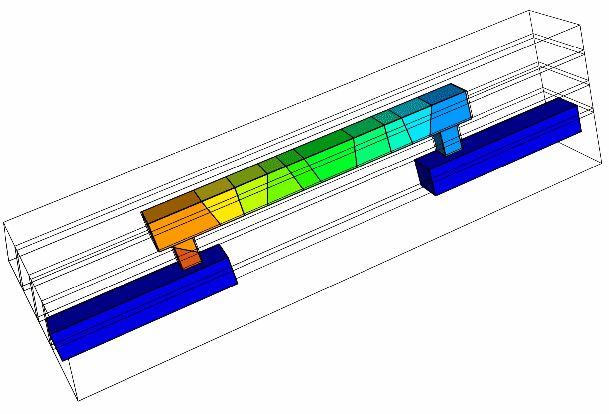Next: 3.1 Electro-Thermal Problem Up: Dissertation R.L. de Orio Previous: 2.6 On the Void

# 3. A General TCAD Electromigration Model

In the previous chapter the various physical phenomena related to electromigration were described and several physically based models were presented. Most of these models, however, are either based on several simplifying assumptions in order to obtain analytical expressions or can only be applied for simulation of simple interconnect geometries. Thus, they cannot cope with the complex modern interconnect structures, as that shown in Figure 3.1. Thus, in this chapter a general electromigration model for implementation in a TCAD tool for simulation of realistic three-dimensional interconnect structures is developed.Since the electromigration simulation requires a multiphysics approach, the model equations can be most conveniently separated in smaller blocks. Starting with the description of the electro-thermal problem, the distributions of electric potential, electric field, current density, and temperature in the interconnect can be determined. Then, the material transport equations are presented. Here, an approach for taking into account the effect of grain boundaries and material interfaces as fast diffusivity paths is described. Moreover, the influence of the mechanical stress on the diffusivity is discussed and the equation for the strain evolution as a result of material transport is derived. The dynamic behavior of grain boundary and material interfaces acting as sites for vacancy generation and annihilation is treated in detail. Then, the mechanical equations which describe the line deformation and the corresponding stress build-up are explained. Finally, a brief model overview is given, where the blocks of equations are presented, which have to be numerically solved for electromigration simulations.

SubsectionsNext: 3.1 Electro-Thermal Problem Up: Dissertation R.L. de Orio Previous: 2.6 On the Void

R. L. de Orio: Electromigration Modeling and Simulation